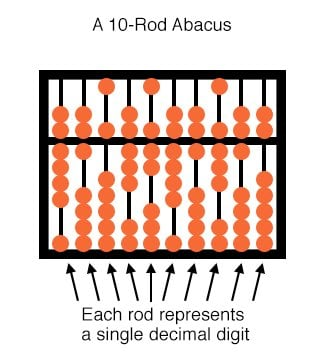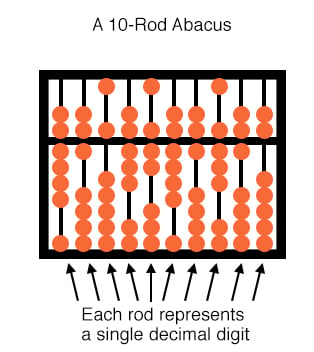## Where did the definitions for bit grouping terms come from?

Definitions for bit grouping terms were taken from Eric S. Raymond’s “Jargon Lexicon,” an indexed collection of terms—both common and obscure—germane to the world of computer programming.

# Bit Grouping

## Chapter 2 - Binary Arithmetic

The singular reason for learning and using the binary numeration system in electronics is to understand how to design, build, and troubleshoot circuits that represent and process numerical quantities in digital form.

Since the bivalent (two-valued) system of binary bit numeration lends itself so easily to representation by “on” and “off” transistor states (saturation and cutoff, respectively), it makes sense to design and build circuits leveraging this principle to perform binary calculations.

If we were to build a circuit to represent a binary number, we would have to allocate enough transistor circuits to represent as many bits as we desire. In other words, in designing a digital circuit, we must first decide how many bits (maximum) we would like to be able to represent, since each bit requires one on/off circuit to represent it.

This is analogous to designing an abacus to digitally represent decimal numbers: we must decide how many digits we wish to handle in this primitive “calculator” device, for each digit requires a separate rod with its own beads.A ten-rod abacus would be able to represent a ten-digit decimal number, or a maximum value of 9,999,999,999. If we wished to represent a larger number on this abacus, we would be unable to, unless additional rods could be added to it.

In digital, electronic computer design, it is common to design the system for a common “bit width:” a maximum number of bits allocated to represent numerical quantities. Early digital computers handled bits in groups of four or eight.

More modern systems handle numbers in clusters of 32 bits or more. To more conveniently express the “bit width” of such clusters in a digital computer, specific labels were applied to the more common groupings.

Eight bits, grouped together to form a single binary quantity, is known as a byte. Four bits, grouped together as one binary number, is known by the humorous title of nibble, often spelled as nybble.

A multitude of terms have followed byte and nibble for labeling specific groupings of binary bits. Most of the terms shown here are informal, and have not been made “authoritative” by any standards group or other sanctioning body.

However, their inclusion into this chapter is warranted by their occasional appearance in technical literature, as well as the levity they add to an otherwise dry subject:

• Bit: A single, bivalent unit of binary notation. Equivalent to a decimal “digit.”
• Crumb, Tydbit, or Tayste: Two bits.
• Nibble, or Nybble: Four bits.
• Nickle: Five bits.
• Byte: Eight bits.
• Deckle: Ten bits.
• Playte: Sixteen bits.
• Dynner: Thirty-two bits.
• Word: (system dependent).

The most ambiguous term by far is word, referring to the standard bit-grouping within a particular digital system. For a computer system using a 32 bit-wide “data path,” a “word” would mean 32 bits.

If the system used 16 bits as the standard grouping for binary quantities, a “word” would mean 16 bits. The terms playte and dynner, by contrast, always refer to 16 and 32 bits, respectively, regardless of the system context in which they are used.

Context dependence is likewise true for derivative terms of word, such as double word and longword (both meaning twice the standard bit-width), half-word (half the standard bit-width), and quad (meaning four times the standard bit-width).

One humorous addition to this somewhat boring collection of word-derivatives is the term chawmp, which means the same as half-word. For example, a chawmp would be 16 bits in the context of a 32-bit digital system, and 18 bits in the context of a 36-bit system. Also, the term gawble is sometimes synonymous with word.

Definitions for bit grouping terms were taken from Eric S. Raymond’s “Jargon Lexicon,” an indexed collection of terms—both common and obscure—germane to the world of computer programming.

RELATED WORKSHEETS:

• Share
Published under the terms and conditions of the Design Science License